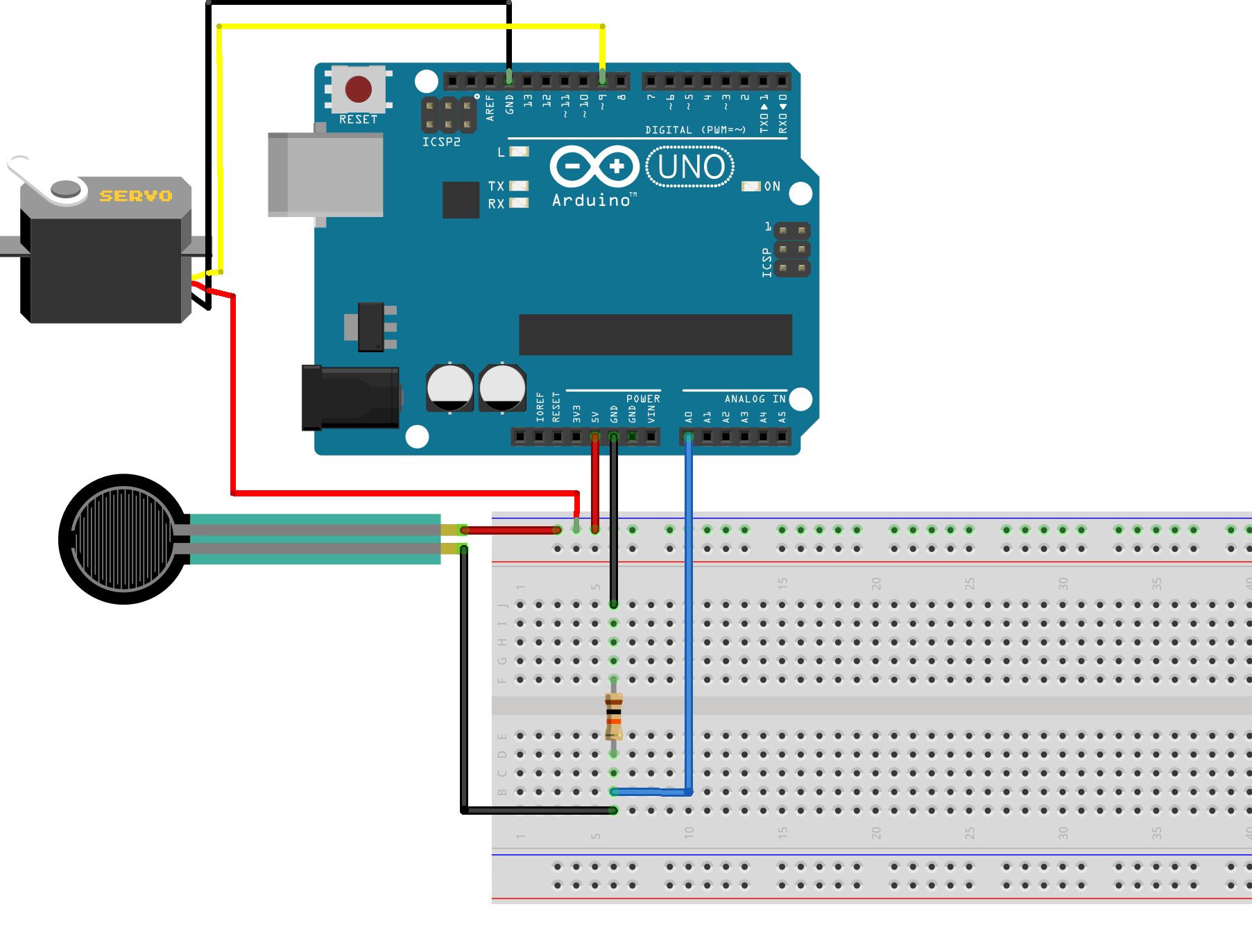Control a Servo with a Force Resistive Sensor on Arduino © GPL3+

The force resistive sensor outputs the applied force to the Arduino, and we move the servo according to the amount of force.

• 20,411 views
• 28 respects

Apps and online services

Introduction

A force resistive sensor changes its resistance based on the physical pressure or weight placed on it. We are using them in conjunction with an Arduino to rotate a servo according to the amount of pressure. It uses the following formula to calculate the output voltage:

Vo = Vcc ( R/(R+FSR) )

Making

Make all the connections and upload the code to the Arduino. After applying force to the sensor you will notice the values in the serial monitor.

Code

Arduino codeC/C++
#include <Servo.h>
Servo servo;

void setup(void) {
Serial.begin(9600);
servo.attach(9); //servo at digital pin 9
servo.write(0); //initial point for servo

}

void loop(void) {
Serial.print("Sensor value = ");

int value = map(reading, 0, 1023, 0, 255);

servo.write(value);

delay(100);
}

SchematicsServo Control with TV Remote Control

Project showcase by eldo85

• 24,567 views
• 48 respects

• 27,364 views
• 79 respects

by Jasleen

• 35,411 views
• 28 respects

Control LED By Clap Using Arduino and Sound Sensor

• 165,083 views
• 96 respects

• 73,520 views